# Use a V Notch Weir to Measure Open Channel Flow Rate

## Introduction

The name for a v notch weir is very descriptive, as you can see in the picture and diagrams in the next couple of sections. A v notch weir is simply a ‘v notch’ in a plate that is placed so that it obstructs an open channel flow, causing the water to flow over the v notch. It is used to meter flow of water in the channel, by measuring the head of water over the v notch crest. The v notch weir is especially good for measuring a low flow rate, because the flow area decreases rapidly as the head over the v notch gets small.

## Sharp Crested Weir Background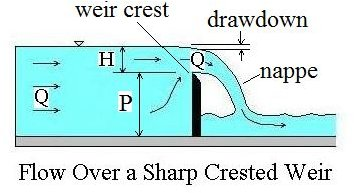The v notch weir is one type of sharp crested weir for which background information is given in Open Channel Flow Measurement 1. Additional general sharp crested weir information is given in this section and then v notch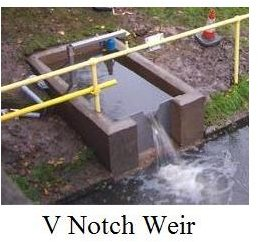weir equations are discussed in the next two sections. The diagram at the left shows some parameters and terminology used with a sharp crested weir for open channel flow rate measurement.

The weir crest is the top of the weir. For a v notch weir it is the point of the notch, which is the lowest point of the weir opening. The term nappe is used for the sheet of water flowing over the weir. The equations to meter flow in this article require free flow, which takes place when there is air under the nappe. The drawdown is the decrease in water level going over the weir due to the acceleration of the water. The head over the weir is shown as H in the diagram; the height of the weir crest is shown as P; and the open channel flow rate or discharge is shown as Q.

## Fully Contracted, 90 Degree, V Notch Weir Equation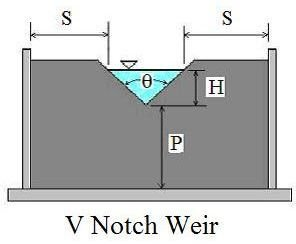The equation recommended by the Bureau of Reclamation in their Water Measurement Manual,for use with a fully contracted, 90o, v notch, sharp crested weir with free flow conditions and 0.2 ft < H < 1.25 ft, is:

Q = 2.49H2.48, where Q is discharge in cfs and H is head over the weir in ft.

The conditions for the v notch weir to be fully contracted are:

P > 2Hmax, S > 2Hmax.

The diagram at the left shows the parameters H, P, θ and S for a v notch weir as used for open channel flow rate measurement.

## V Notch Weir Equation for Notch Angle Other than 90 Degrees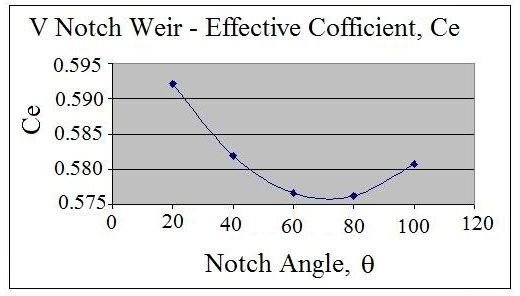For notch angles other than 90o, the Kindsvater-Carter equation, as given below, from the Bureau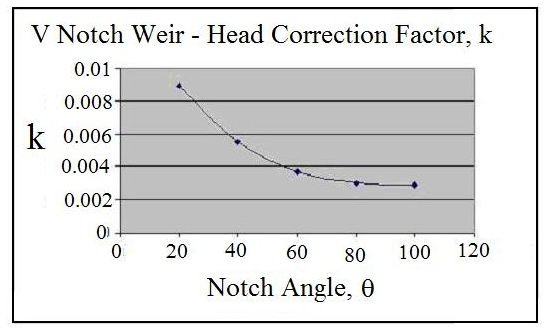of Reclamation, Water Measurement Manual, should be used. That equation is: Q = 4.28 Ce Tan(θ/2)(H + k)5/2, where Q and H are as previously defined, θ is the angle of the v notch, Ce is the effective discharge coefficient, and k is a head correction factor. The diagram at the left is a graph of Ce as a function of notch angle, θ, and the diagram at the right gives k as a function of θ.

## Example Calculation

Problem Statement: Calculate the minimum flow rate and maximum flow rate covered by the range of 0.2 ft to 1.25 ft for the head over a v notch weir that is fully contracted. (Note: In order to be fully contracted, P and S must both be greater than 2Hmax, that is greater than (2)(1.25) or greater than 2.5 ft.)

Solution: Substituting the given values of H into the equation, Q – 2.49 H2.48, gives:

Qmin = (2.49)(0.22.48) = 0.046 cfs

Qmax = (2.49)(1.252.48) = 4.33 cfs

For Excel spreadsheet templates that you can download to make V notch weir calculations, see the article, "Use of Excel Spreadsheet Templates for V-Notch Weir Calculations."

## References

1. U.S. Dept. of the Interior, Bureau of Reclamation, 2001 revised, 1997 third edition, Water Measurement Manual, available for online use or download at: https://www.usbr.gov/pmts/hydraulics_lab/pubs/wmm/index.htm.

2. Bengtson, Harlan H., Open Channel Flow III – Sharp Crested Weirs, an online continuing education course for PDH credit, https://www.online-pdh.com/engcourses/course/view.php?id=87

3. Munson, B. R., Young, D. F., & Okiishi, T. H., Fundamentals of Fluid Mechanics, 4th Ed., New York: John Wiley and Sons, Inc, 2002.

## This post is part of the series: Open Channel Flow Measurement

Open channel flow rate measurement is usually done by measuring a change in water depth. It can be done with a weir or flume. Common types are the sharp crested weir (including V-notch weir, rectangular weir, and cipolletti weir), the broad crested weir, the Parshall flume and venturi flume.